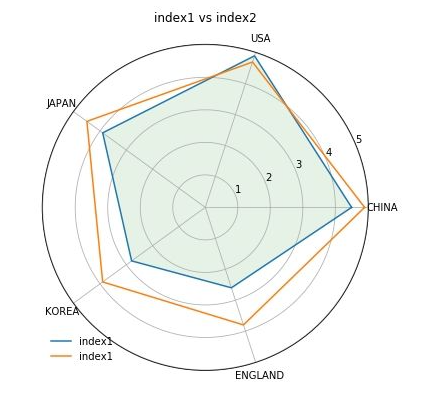python如何画极坐标雷达图

#生成数据，注意第一组与最后一组数据是相同的，确保可以连成一个闭合多边形
country = ["CHINA", "USA", "JAPAN", "KOREA", "ENGLAND"]
index1= [4.5, 4.9, 3.9, 2.8, 2.6, 4.5]
index2= [4.9, 4.7, 4.5, 3.9, 3.8, 4.9]
plt.figure(figsize = (10, 6)) #设置图形大小
plt.subplot(polar = True) #设置图形为极坐标图
theta = np.linspace(0, 2 * np.pi, len(index1)) #根据index1的数量将圆均分
#设置网格，标签
lines, labels = plt.thetagrids(range(0, 360, int(360/len(country))), (country))
#绘制index1
plt.plot(theta,index1 )
plt.fill(theta,index1 , 'g', alpha=0.1) #设置颜色与透明度
#绘制index2
plt.plot(theta, index2)

# 添加图例和标题
plt.legend(labels=('index1', 'index2'), loc = 'best',frameon = True) # loc为图例位置
plt.title("index1 vs index2")；Python开发开发项目